## 3.3.3 Data definition

Consider a 2D mesh which will either serve as the lower section, or be used to define this section.

One section being formed, we can deduce other sections from it. The layers of 3D elements will then be formed by connecting the sections defined previously, two-by-two.

Let:

• M be a a point in the 3D mesh,
• X, Y and Z its coordinates,
• m the 2D generic point from which point M was constructed, and
• x and y its coordinates.

The basis, or lower section, is section 0, the sections being numbered from 0 to n. The layers are numbered from 1 to n, layer i linking sections i-1 and i. Furthermore, vertical, horizontal, etc., items are called so with reference to a classical cylinder positioned on the plane z=0, thus relative to the topology of the domain.

### Construction of the sections

• Construction of the base: There are two possibilities (option BFONC).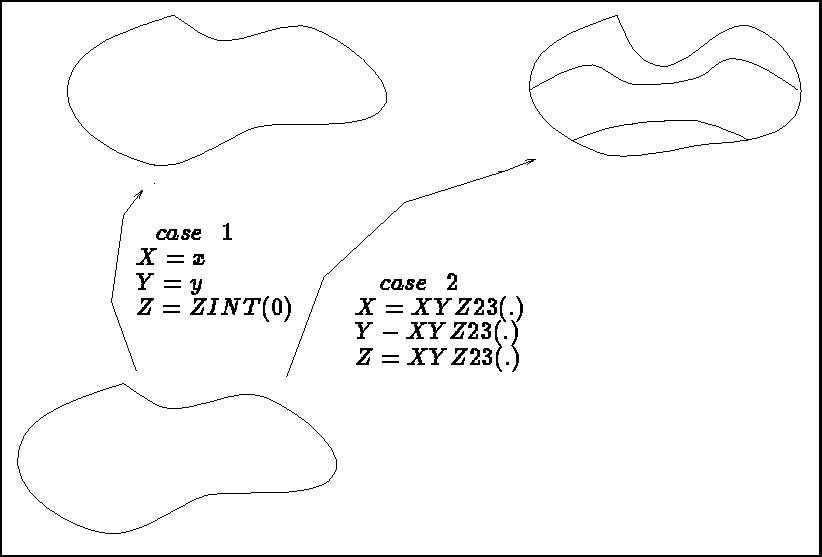Figure 3.9: The basis as the image of the 2D mesh (2 cases)

• BFONC = .FALSE. The base is the 2D mesh with side given: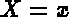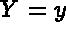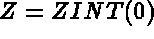• BFONC = .TRUE. The base is the result of a transformation of the 2D mesh via a function: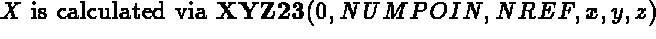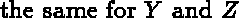• Construction of sections 1 to n: several possibilities (TTYPE).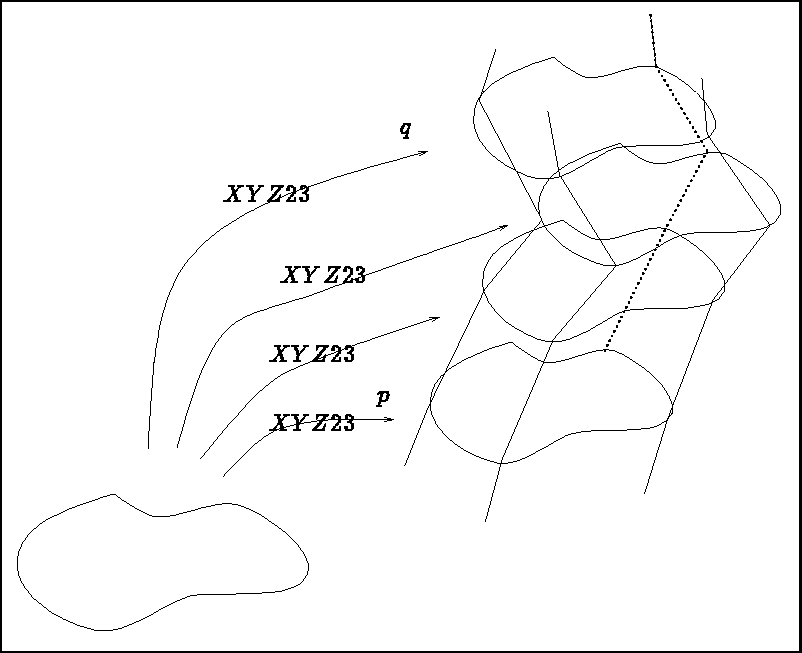Figure 3.10: Sections p to q as the image of the 2D mesh

• TTYPE = -2 Total definition of sections p to q from a 2D mesh. The sections p to q result from a transformation of the 2D mesh via a function, thus for section i: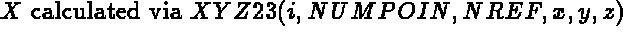• Global definition of k sections. Two cases are possible:
• construction from the data of a section and definition of the k sections by translation of the section given;
• construction of the k sections by interpolation between a first section already created and a section defined presently via function XYZ23 (thus this section is a transformation of a 2D generic mesh).

We therefore have 3 possibilities in this case:

• TTYPE = - 4 Starting with an existing section, the k sections are deduced by translation according to the cylindrical axis (according to the z axis, topologically speaking) with step-size given in array ZINT(.).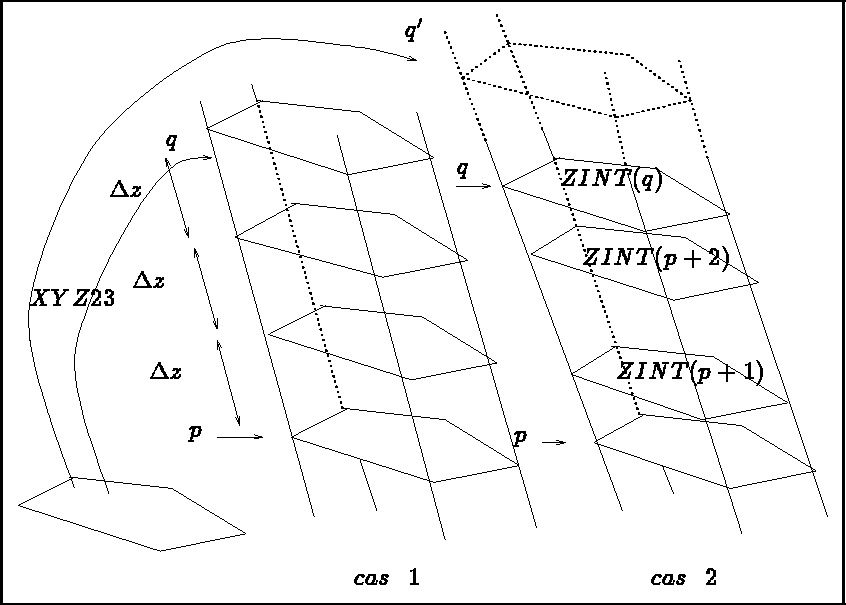Figure 3.11: Sections p to q as interpolated between 2 sections (2 cases)

• TTYPE = - 5 The k sections result from an interpolation between two extreme sections with indices p and q. Section p exists already and section q is defined as the transformation of the 2D generic mesh via function XYZ23. The sections created with indices p+1 to q are interpolated between sections p and q with a constant step in z (from a topological point of view).

• TTYPE = - 6 The k sections result from an interpolation between two extreme sections with indices p and q'. Section p exists already and section q' is defined as the transformation of the 2D generic mesh via function XYZ23. The sections created with indices p+1 to q are interpolated between sections p and q' as a function of a variable step in z (from a topological point of view) given in array ZINT(.) with indices p+1, p+2, ..., q. Note that q and q' may be equal, or not (in this case the upper section is virtual and is only useful during the construction process).

• Local definition of section i starting from section i-1. Two possibilities are encountered: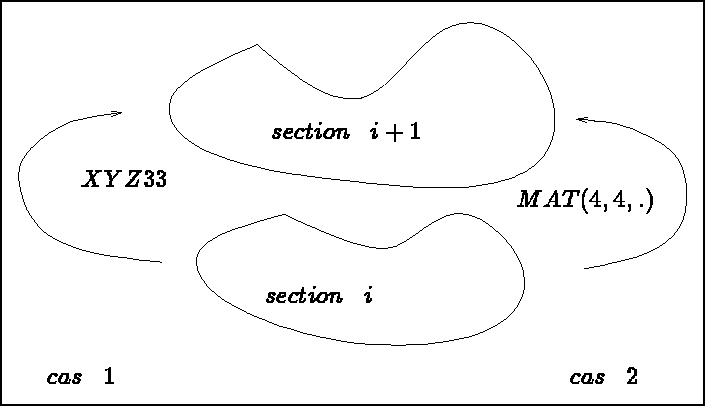Figure 3.12: Section i as the image of section i-1 (2 cases)

• TTYPE = -3 Section i results from the transformation of section i-1 via function XYZ33, i.e: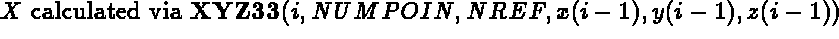• TTYPE = -1 The same case with a transformation defined by its matrix MAT(4,4,i).

Summary: The basis is constructed like a 2D mesh with a side given, or like the transformations of a 2D mesh via XYZ23.

The sections are deduced from the 2D mesh via XYZ23, interpolated between two extreme sections with sides equidistant or given, the one deduced from the other, step by step, via XYZ33 or MAT.

The different methods could also be combined.

### Construction of the layers

One layer is defined by 2 consecutive sections. The constituent elements of the layer are created by connecting the corresponding points. In practice, at the time that at least 2 sections have been created, we create at least 1 layer in parallel.

### Options

It is possible to identify the points, edges and faces of the basis and the upper section of the cylinder for the case where the latter is closed (option COLLER).

Certain transformations (for example rotation) can generate degenerate elements (when the rotational axis is supported by an edge of the initial mesh). In this case, the elements created are no longer the natural correspondents of the 2D elements (an automatic operation performed by the module).

Certain transformations (for example rotation) can generate degenerate elements (when the rotational axis is internal to the mesh). In this case, the elements created are no longer the natural correspondents of the 2D elements (option RAPIDE).

### The physical attributes

The physical attributes (sub-domains and references) of the 3D mesh are generated using the numbers present in the initial 2D mesh. This correspondence indicates:

• the limits of action: for example, from section i to section j
• the type of transfer: for example, the numbers of the vertical edges are deduced from the numbers of the 2D points
• the correspondence between the numbers: for example,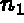becomes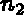Let NBDES be the number of descriptions to assign, then
for I=1,NBDES we give for five values: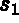,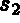, TYPE,,defined in array DESREF(5,NBDES) by:

• value 1: the index of the starting section ()
• value 2: the index of the arrival section ()
• value 3: the type of transfer (parameter TYPE)
• 1: 2D sub-domain number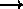3D sub-domain number
• 2: 2D sub-domain number3D horizontal faces reference
• 3: 2D edge references3D vertical faces reference

• 4: 2D edge references3D horizontal edges reference
• 5: 2D edge references3D horizontal edges and vertical faces reference
• 6: 2D point reference3D vertical edges reference
• 7: 2D point reference3D points reference
• 8: 2D point reference3D points and vertical edges reference
• 0: assignment of a number () to all the items (faces, edges and points) of a given section ()
• -1: direct correspondence of the 2D references (points and edges) to the 3D items of the vertical faces
• value 4: the 2D number serving as reference ()
• value 5: the 3D number () to associate to the items 3D deduced from the 2D items having the above number,, as attribute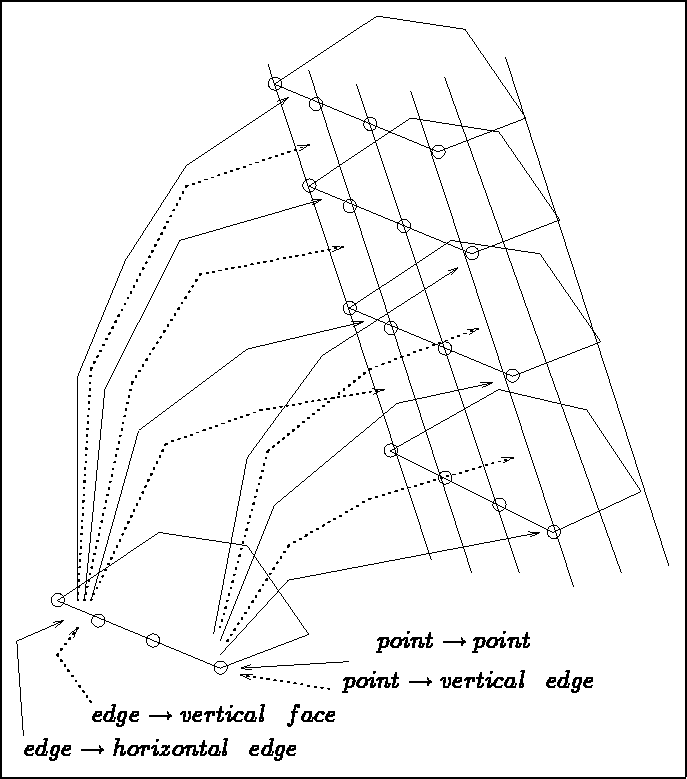Figure 3.13: The transfers and physical attributes

Note that, in the same way, we can completely define all the items of the 3D mesh: points, sides and faces whatever their position may be (see figure 3.13).

Important remark: The assignments are done in the order of their description (there is no commutation; a request given supersedes a request prescribed beforehand).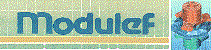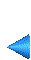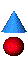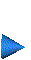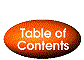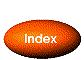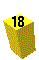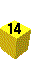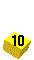Next: 3.3.4 Calling of module MA2D3E Up: 3.3 Extrusion method (cylindrical topology) Prev: 3.3.2 Simplified version Index Contents